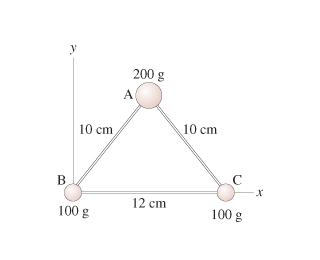# Problem: Find the moment of inertia about an axis that passes through mass A and is perpendicular to the page.Express your answer to two significant figures and include the appropriate units.

###### FREE Expert Solution

Moment of inertia of a point mass, located a distance, r, from the axis of rotation is

$\overline{){\mathbf{I}}{\mathbf{=}}{\mathbf{m}}{{\mathbf{r}}}^{{\mathbf{2}}}}$

rB = rC = 10 cm(1m/100 cm) = 0.10 m

84% (228 ratings)###### Problem DetailsFind the moment of inertia about an axis that passes through mass A and is perpendicular to the page.

Express your answer to two significant figures and include the appropriate units.